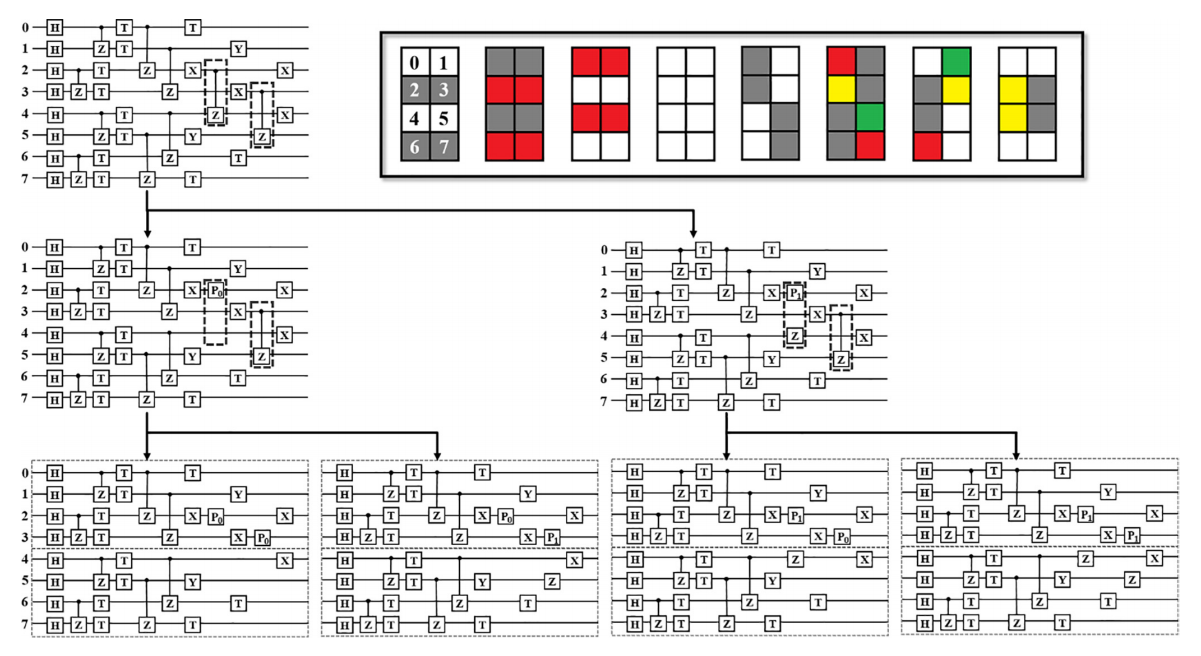# 部分振幅量子虚拟机¶## 使用介绍¶

QPanda2中设计了 PartialAmplitudeQVM 类用于运行部分振幅模拟量子计算，同时提供了相关接口，它的使用很简单。

auto machine = new PartialAmplitudeQVM();


machine->init();


auto prog = QProg();
auto qlist = machine->qAllocMany(10);

for_each(qlist.begin(), qlist.end(), [&](Qubit *val) { prog << H(val); });
prog << CZ(qlist, qlist) << CZ(qlist, qlist) << CZ(qlist, qlist);
...
machine->run(prog);


machine->run("D:\\OriginIR");


## 实例¶

#include "QPanda.h"
USING_QPANDA

int main(void)
{
auto machine = new PartialAmplitudeQVM();
machine->init();
auto qlist = machine->qAllocMany(10);

// 构建量子程序
auto prog = QProg();
for_each(qlist.begin(), qlist.end(), [&](Qubit *val) { prog << H(val); });
prog << CZ(qlist, qlist)
<< CZ(qlist, qlist)
<< CZ(qlist, qlist)
<< RZ(qlist, PI / 4)
<< RX(qlist, PI / 4)
<< RX(qlist, PI / 4)
<< RY(qlist, PI / 4)
<< CZ(qlist, qlist)
<< RZ(qlist, PI / 4)
<< RZ(qlist, PI / 4)
<< CZ(qlist, qlist)
<< RY(qlist, PI / 4)
<< RZ(qlist, PI / 4)
<< CR(qlist, qlist, PI / 2);

// 获取量子态所有分量的振幅
machine->run(prog);
auto res = machine->getQState();

// 打印特定量子态分量的振幅
cout << res["0000000000"] << endl;
cout << res["0000000001"] << endl;

machine->finalize();
}


(-0.00647209,-0.00647209)
(8.5444e-18,-0.00915291)
...


• PMeasure(std::string) ,输入的参数表示获取测量所有比特构成量子态的概率的结果集的前多少项，比如如下例子，我们获取所有量子态的概率分布结果的前6项，程序运行如下：

auto res = machine->PMeasure("6");
for (auto val :res)
{
std::cout << val.first << " : " << val.second << std::endl;
}


结果输出如下，每个结果的序号表示量子态的下标，后面的值表示概率：

0 : 8.37758e-05
1 : 8.37758e-05
2 : 8.37758e-05
3 : 8.37758e-05
4 : 0.000488281
5 : 0.000488281

• PMeasure(QVec,std::string) ,输入的第一个参数表示选取哪几个量子比特构成的量子态的概率，第二个参数表示选取结果的前多少项，使用示例如下：

QVec qv = { qlist,qlist,qlist ,qlist ,qlist ,qlist ,qlist ,qlist,qlist };
auto res2 = machine->PMeasure(qv, "6");

for (auto val :res)
{
std::cout << val.first << " : " << val.second << std::endl;
}


结果输出如下，每个结果的序号表示量子态的下标，后面的值表示概率：

0 : 0.000167552
1 : 0.000167552
2 : 0.000976562
3 : 0.000976562
4 : 0.000976562
5 : 0.000976562

• getProbDict(qvec,std::string) ,输入的第一个参数表示选取哪几个量子比特构成的量子态的概率，第二个参数表示选取结果的前多少项，使用示例如下：

QVec qvec;
for_each(qlist.begin(), qlist.end(), [&](Qubit *val) { qvec.emplace_back(val); });

auto res = machine->getProbDict(qvec,6);
for (auto val :res)
{
std::cout << val.first << " : " << val.second << endl;
}


结果输出如下，每个结果的前半部分表示量子态的二进制形式，后面的值表示概率：

0000000000 : 8.37758e-05
0000000001 : 8.37758e-05
0000000010 : 8.37758e-05
0000000011 : 8.37758e-05
0000000100 : 0.000488281
0000000101 : 0.000488281

• pMeasureBinIndex(std::string) ,输入的参数表示指定需要测量的量子态二进制形式，使用示例如下：

auto res = machine->pMeasureBinIndex("0000000001");
std::cout << res << std::endl;


结果输出如下，表示目标量子态的概率值：

8.37758e-05

• pMeasureDecIndex(std::string) ,输入的参数表示指定需要测量的量子态十进制下标形式，使用示例

auto res = machine->pMeasureBinIndex("1");
std::cout << res << std::endl;


结果输出如下，表示目标量子态的概率值：

8.37758e-05

• pMeasureSubset(QProg &, std::vector<std::string>) ,输入的第一个参数表示待运行的量子线路，第二个参数表示需要测量的量子态二进制下标形式构成的子集，使用示例如下：

std::vector<std::string> set = { "0000000000","0000000001","0000000100" };
auto res = machine->PMeasureSubset(prog, set);

for (auto val : res)
{
std::cout << val.first << " : " << val.second << endl;
}


结果输出如下：

0000000000 : 8.37758e-05
0000000001 : 8.37758e-05
0000000100 : 0.000488281


警告

1. 部分接口，比如 getQState()PMeasure(std::string)PMeasure(QVec,std::string)pMeasureBinIndex(std::string) 以及 pMeasureDecIndex(std::string) 等会在后续的版本中舍弃。
2. 部分振幅虚拟机会保留 pMeasureSubset(QProg &, std::vector<std::string>) 接口。